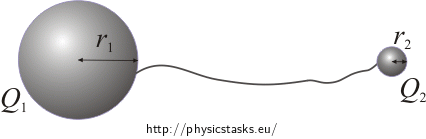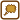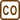## Electrically connected charged balls

Two balls with radius r1 = 4 cm and radius r2 = 1 cm are connected by a long thin wire. How is the electric charge divided between them?• #### Hint

What do we know about the potential difference between the balls when they are connected by a conductor?

• #### Hint: Ball

When the balls are connected by a conductive wire, the charge can move from one ball to other. The ball with higher potential will be partly discharged trough the wire while charging the other ball. At the end of this proces, both balls’ potentials will be the same.

Charged ball’s potential is in direct proportion to the charge of the ball and in reciprocal proportion to the ball’s radius.

• #### Analysis

When two charged balls are connected, the charge will move from one ball to another until the potentials of both balls are equal. We can evaluate the potential on each ball (as a function of charge on the ball) and set the potentials equal to each other. This provides us an equation. Afterwards we can obtain the proportion of charges on several balls by modifying that equation.

Both balls are conductive, therefore all the charge will be placed on the surface of the balls. The potential outside the ball and on the surface of the ball is same as the potential around a point charge. The potential is directly proportional to the charge and inversely proportional to the radius of the ball (or distance from the point charge).

The density of the charge on the surface of the ball is the total charge on the ball divided by the surface area (surface area of the ball can be evaluated as a function of the radius). Afterwards we have to compare the charge densities of the balls.

• #### Charges ratio calculation

When two objects are electrically connected, the charge distributes so that the potential of both objects is the same.

There is the same formula for the potential out of the ball and on the surface of the ball. This formula is the same as the formula of potential around a point charge:

$\varphi \, =\,k\,\frac{Q}{r}$

So the potential of the first ball is: $$\varphi_1 \, =\,k\,\frac{Q_1}{r_1}$$,

and the potential of the second ball is: $$\varphi_2 \, =\,k\,\frac{Q_2}{r_2}$$.

The potentials of both balls are the same.

$\varphi_1\,=\,\varphi_2$ $k\,\frac{Q_1}{r_1}\, =\,k\,\frac{Q_2}{r_2}$

Let’s reduce the constant k and express the ratio of the charges. (We have to convert both charges on the same side of the equation.)

$\frac{Q_1}{Q_2}\,=\,\frac{r_1}{r_2}$

The charge is distributed in the same ratio as the ratio of balls radii.

That means there is more charge on the surface of the bigger ball.

• #### Calculation of the ratio of surface charge density

The surface charge density on the ball depends on the total charge on the ball and the ball’s surface.

$Q\,=\,\sigma S$

Let’s substitute the formula for the surface of a sphere S = 4πr2, to get the formulas for surface charge densities on the balls:

$Q_1\,=\,\sigma_1 4\pi r_1^2 \hspace{20px} \Rightarrow \sigma_1\,=\,\frac{Q_1} {4\pi r_1^2}$ $Q_2\,=\,\sigma_24\pi r_2^2 \hspace{20px} \Rightarrow \sigma_2\,=\,\frac{Q_2} {4\pi r_2^2}$

Now we can calculate the ratio of surface charge densities on the balls.

$\frac{\sigma_1}{\sigma_2}\,=\,\frac{\frac{Q_1} {4\pi r_1^2}}{\frac{Q_2} {4\pi r_2^2}}$

Let’s modify the compound

$\frac{\sigma_1}{\sigma_2}\,=\,\frac{Q_1 4\pi r_2^2}{Q_24\pi r_1^2}\, ,$

and reduce constant 4π.

$\frac{\sigma_1}{\sigma_2}\,=\,\frac{Q_1 r_2^2}{Q_2 r_1^2} \tag{*}$

In previous part of solution (Charges ratio calculation) we found that the ratio of charges on the balls is the same as the ratio of radii of these balls $\frac{Q_1}{Q_2}\,=\,\frac{r_1}{r_2}$ . We can substitute that formula into the formula (*).

$\frac{\sigma_1}{\sigma_2}\,=\,\frac{r_1 r_2^2}{r_2 r_1^2}$

By reducing r1 and r2 we get the final formula for the ratio of surface densities of charges.

$\frac{\sigma_1}{\sigma_2}\,=\,\frac{r_2}{r_1}$

The ratio of surface charge densities is equal to the inverted ratio of the balls radii.

That means there is lower density on the bigger ball.

• #### Solution by consideration

If we electrically connect two balls, the charge on the balls would redistribute, so the potential on the both balls is the same.

The potential on the ball is the same as the point charge potential. It is directly proportional to the charge and inversely proportional to the ball radius. The bigger ball radius is 4 times bigger than the radius of smaller one, so there must be 4 times more charge on the bigger ball when the potential of both balls is the same.

The charge on the bigger ball is 4 times bigger. The radius of the bigger ball is 4 times bigger, so the surface is 16 times larger than the surface of the smaller ball. We can get the surface charge density by dividing the charge on the ball by the surface area. So the surface charge density is 4 times lower on the surface of the bigger ball than on the surface of the smaller one $$\left(\frac{16}{4}\,=\,4\right)$$.

• #### Calculation

$r_1\,=\,4\,\mathrm{cm}$ $r_2\,=\,1\,\mathrm{cm}$ $\frac{Q_1}{Q_2}\,=\,?$ $\frac{\sigma_1}{\sigma_2}\,=\,?$

Note: There is the ratio of radii in the formula, so we don’t need to use base units in radii enumeration, using the same units is enough.

$\frac{Q_1}{Q_2}\,=\,\frac{r_1}{r_2}\,=\,\frac{4}{1}$ $\frac{\sigma_1}{\sigma_2}\,=\,\frac{r_2}{r_1}\,=\,\frac{1}{4}$

The charge on the bigger ball is 4 times bigger, but the surface charge density is 4 times lower than on the smaller ball.

The charge is divided in the same ratio as the ratio of balls radii $$\frac{Q_1}{Q_2}\,=\,\frac{r_1}{r_2}\,=\,\frac{4}{1}$$.
The ratio of the areal densities of the charge is equal to the inverted ratio of balls radii $$\frac{\sigma_1}{\sigma_2}\,=\,\frac{r_2}{r_1}\,=\,\frac{1}{4}$$.